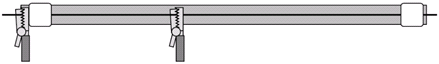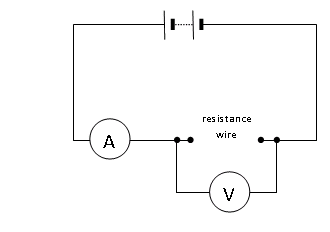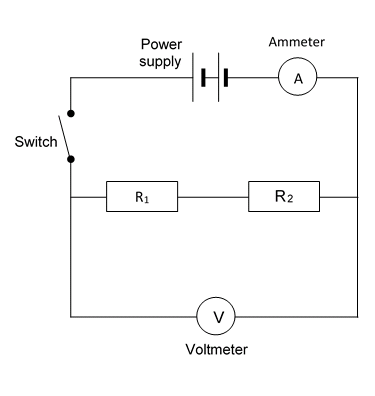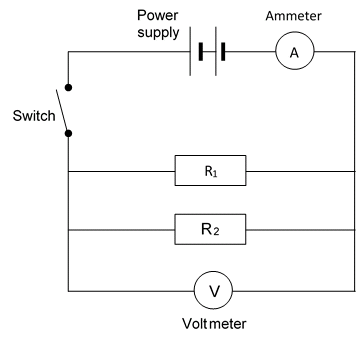# Investigating Resistors

## Experimental Set Up

There are two experiments in this required practical.

Both are centred around factors that affect resistance in a circuit. The two are:

1. The length of a wire at a constant temperature
2. Combinations of resistors in series and parallel

## Experiment One Method

Method

You are provided with the following:

• A battery or suitable power supply
• Ammeter or multimeter
• Voltmeter or multimeter
• Crocodile clips
• Resistance wire eg constantan of different diameters attached to a metre ruler

Read these instructions carefully before you start work.

1. Connect the circuit. It may be helpful to start at the positive side of the battery or power supply. This may be indicated by a red socket.
2. Connect a lead from the red socket to the positive side of the ammeter.
3. Connect a lead from the negative side of the ammeter (this may be black) to the crocodile clip at the zero end of the ruler.1. Connect a lead from the other crocodile clip to the negative side of the battery. The main loop of the circuit is now complete. Use this lead as a switch to disconnect the battery between readings.
2. Connect a lead from the positive side of the voltmeter to the crocodile clip the ammeter is connected to.
3. Connect a lead from the negative side of the voltmeter to the other crocodile clip.1. Record on a table the:
• Length of the wire between the crocodile clips
• The readings on the ammeter
• The readings on the voltmeter.

You will need four columns in total. Length of wire (cm) Potential difference (V) Current (A) Resistance (Ω)

1. Move the crocodile clip and record the new ammeter and voltmeter readings. Note that the voltmeter reading may not change. Repeat this to obtain several pairs of meter readings for different lengths of wire.
2. Calculate and record the resistance for each length of wire using the equation:

resistance in (Ω) = potential difference (V) / current in (A)

1. Plot a graph with:
• ‘Resistance in ’ on the y-axis
• ‘Length of wire in cm’ on the x-axis.
2. You should be able to draw a straight line of best fit although it may not go through the origin.

Can you account for the extra resistance?

## Experiment One Results

In the results a clear trend/pattern will appear. It is clear to see that as the length of the wire increases, so does the resistance. This is because there are more ions for the electrons to hit, therefore more collision and a higher resistance.

## Experiment Two Method

1. Connect the circuit for two resistors in series, as shown in the diagram.
2. Switch on and record the readings on the ammeter and the voltmeter.
3. Use these readings to calculate the total resistance of the circuit.
4. Now set up the circuit for two resistors in parallel.
5. Switch on and record the readings on the ammeter and the voltmeter.
6. Use these readings to calculate the total resistance of the circuit.
7. With one single resistor in the circuit, the total resistance would be 10 ohms. What is the effect on the total resistance of adding:
8. another identical resistor in series
9. another identical resistor in parallel?
10. You could also try setting up a circuit with three resistors in series and one with three resistors in parallel.
11. What conclusions can you come to about the effect of adding resistors
12. In series
13. In parallel1.## Experiment Two Results

Like experiment one, the results for experiment two will also be extremely clear.

There is more resistance for resistors in series than in parallel.

The reason why is that in series each electron has to go through every resistor, thus making it high.

In a parallel circuit, because electrons do not go through each resistor, the resistance is lower.

You do not need to be able to calculate the resistance for resistors in parallel. For series it is a simple calculation - just add the resistors together!

What happens to the resistance in a wire as the length is increased?